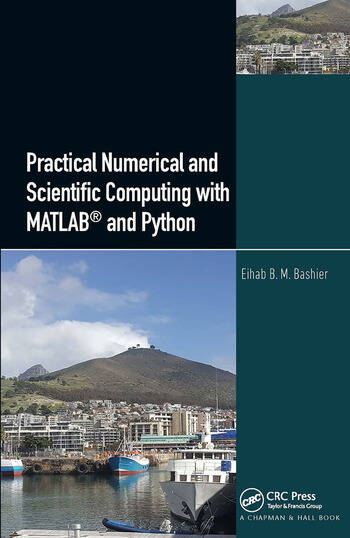# Practical Numerical and Scientific Computing with MATLAB® and Python

## 1st Edition

Eihab B. M. Bashier

CRC Press
Published March 11, 2020
Reference - 330 Pages - 56 B/W Illustrations
ISBN 9780367076696 - CAT# K405798

For Instructors Request Inspection Copy

was \$99.95

USD\$79.96

SAVE ~\$19.99

FREE Standard Shipping!

## Preview

### Summary

Practical Numerical and Scientific Computing with MATLAB® and Python concentrates on the practical aspects of numerical analysis and linear and non-linear programming. It discusses the methods for solving different types of mathematical problems using MATLAB and Python. Although the book focuses on the approximation problem rather than on error analysis of mathematical problems, it provides practical ways to calculate errors.

The book is divided into three parts, covering topics in numerical linear algebra, methods of interpolation, numerical differentiation and integration, solutions of differential equations, linear and non-linear programming problems, and optimal control problems.

This book has the following advantages:

• It adopts the programming languages, MATLAB and Python, which are widely used among academics, scientists, and engineers, for ease of use and contain many libraries covering many scientific and engineering fields.

• It contains topics that are rarely found in other numerical analysis books, such as ill-conditioned linear systems and methods of regularization to stabilize their solutions, nonstandard finite differences methods for solutions of ordinary differential equations, and the computations of the optimal controls. It provides a practical explanation of how to apply these topics using MATLAB and Python.

• It discusses software libraries to solve mathematical problems, such as software Gekko, pulp, and pyomo. These libraries use Python for solutions to differential equations and static and dynamic optimization problems.

• Most programs in the book can be applied in versions prior to MATLAB 2017b and Python 3.7.4 without the need to modify these programs.

This book is aimed at newcomers and middle-level students, as well as members of the scientific community who are interested in solving math problems using MATLAB or Python.

#### Instructors

We provide complimentary e-inspection copies of primary textbooks to instructors considering our books for course adoption.